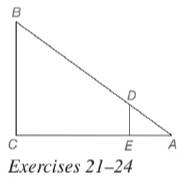Chapter 5.2, Problem 21EElementary Geometry For College St...

7th Edition
Alexander + 2 others
ISBN: 9781337614085

Solutions

Chapter
SectionElementary Geometry For College St...

7th Edition
Alexander + 2 others
ISBN: 9781337614085
Textbook Problem

In Exercises 21 to 24, △ A D E ∼ △ A B C . Given: D E = 4 , A E = 6 , E C = B C Find: B CTo determine

To calculate:

The value of the side BC.

Explanation

Given:

It is given that ΔADEABC.

DE=4,AE=6,andEC=BC

Definition Used:

Similarity between Two polygons:

Two polygons are similar if and only if when all pairs of corresponding angles are congruent and all pairs of corresponding sides are proportional.

If C is a point of XY¯ and XCY, then XC+CY=XY.

Calculation:

According to the definition of similar polygon, ACAE=BCDE=ABAD

Still sussing out bartleby?

Check out a sample textbook solution.

See a sample solution

The Solution to Your Study Problems

Bartleby provides explanations to thousands of textbook problems written by our experts, many with advanced degrees!

Get Started

Find a point on the y-axis that is equidistant from (5, 5) and (1, 1).

Single Variable Calculus: Early Transcendentals, Volume I

Find the domain of the function. a. f(x) = 9x b. f(x) = x+32x2x3

Applied Calculus for the Managerial, Life, and Social Sciences: A Brief Approach

Evaluate the integral. 27. tan3xsecxdx

Single Variable Calculus: Early Transcendentals